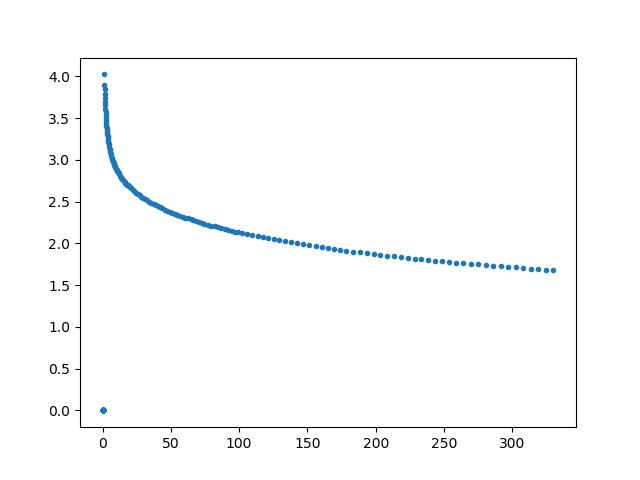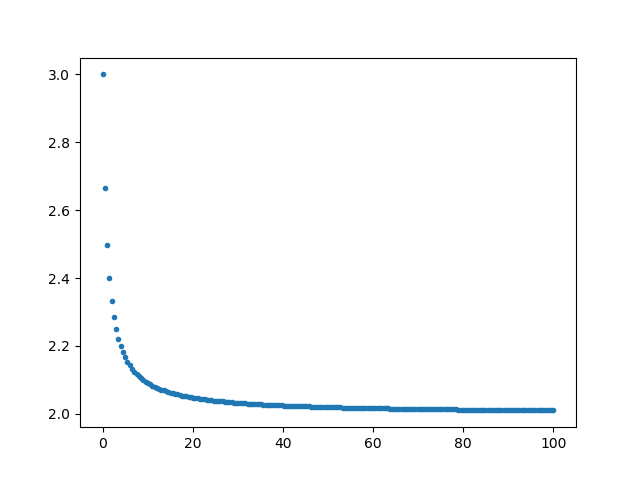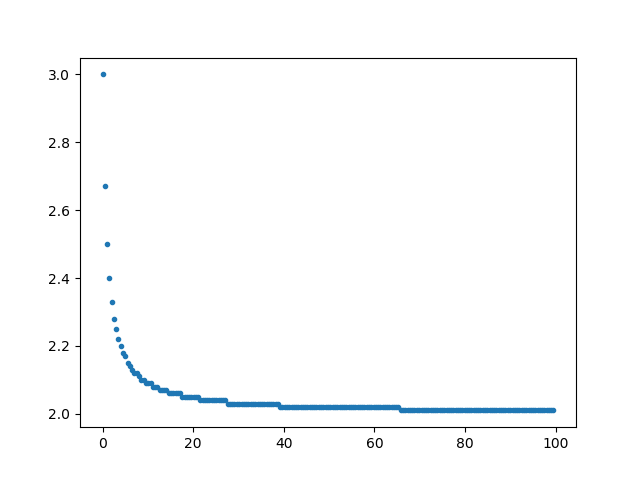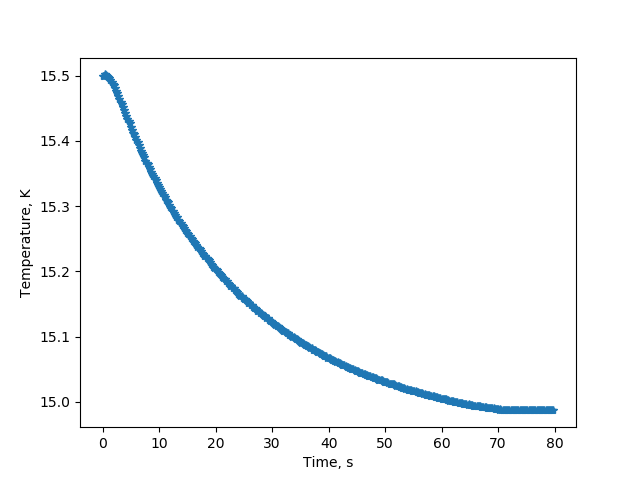This page was generated from docs/examples/driver_examples/Qcodes example with Lakeshore 325.ipynb. Interactive online version:.

# QCoDeS Example with Lakeshore 325

Here provided is an example session with model 325 of the Lakeshore temperature controller

:

%matplotlib notebook

:

import matplotlib.pyplot as plt
import numpy as np

from qcodes.instrument_drivers.Lakeshore import LakeshoreModel325

:

lake = LakeshoreModel325("lake", "GPIB0::12::INSTR")

Connected to: LSCI 325 (serial:LSA2251, firmware:1.8/1.1) in 1.30s


## Sensor commands

:

# Check that the sensor is in the correct status
lake.sensor_A.status()

:

'OK'

:

# What temperature is it reading?
lake.sensor_A.temperature()

:

23.102

:

lake.sensor_A.temperature.unit

:

'K'

:

# We can access the sensor objects through the sensor list as well
assert lake.sensor_A is lake.sensor


## Heater commands

:

# In a closed loop configuration, heater 1 reads from...
lake.heater_1.input_channel()

:

'A'

:

lake.heater_1.unit()

:

'Kelvin'

:

# Get the PID values
print("P = ", lake.heater_1.P())
print("I = ", lake.heater_1.I())
print("D = ", lake.heater_1.D())

P =  400.0
I =  40.0
D =  10.0

:

# Is the heater on?
lake.heater_1.output_range()

:

'Off'


:

curve = lake.sensor_A.curve

:

curve_data = curve.get_data()

:

curve_data.keys()

:

dict_keys(['Temperature (K)', 'log Ohm'])

:

fig, ax = plt.subplots()
ax.plot(curve_data["Temperature (K)"], curve_data['log Ohm'], '.')
plt.show():

curve.curve_name()

:

'CX-1050-SD-HT-1'

:

curve_x = lake.curve

:

curve_x_data = curve_x.get_data()

:

curve_x_data.keys()

:

dict_keys(['Temperature (K)', 'log Ohm'])

:

temp = np.linspace(0, 100, 200)
new_data = {"Temperature (K)": temp, "log Ohm": 1/(temp+1)+2}

fig, ax = plt.subplots()
ax.plot(new_data["Temperature (K)"], new_data["log Ohm"], '.')
plt.show():

curve_x.format("log Ohm/K")
curve_x.set_data(new_data)

:

curve_x.format()

:

'log Ohm/K'

:

curve_x_data = curve_x.get_data()

:

fig, ax = plt.subplots()
ax.plot(curve_x_data["Temperature (K)"], curve_x_data['log Ohm'], '.')
plt.show()## Go to a set point

:

import time

import numpy
from IPython.display import display
from ipywidgets import widgets
from matplotlib import pyplot as plt

"""
Live plot the temperature reading from a Lakeshore sensor channel

Args:
Lakeshore channel object to read the temperature from
time in seconds between two reads of the temperature
total number of reads to perform
"""

# Make a widget for a text display that is contantly being updated
text = widgets.Text()
display(text)

fig, ax = plt.subplots(1)
line, = ax.plot([], [], '*-')
ax.set_xlabel('Time, s')
fig.show()
plt.ion()

# Update the text field

# Add new point to the data that is being plotted

ax.relim()  # Recalculate limits
ax.autoscale_view(True, True, True)  # Autoscale
fig.canvas.draw()  # Redraw

:

lake.heater_1.control_mode("Manual PID")
lake.heater_1.output_range("Low (2.5W)")
lake.heater_1.input_channel("A")
# The following seem to be good settings for our setup
lake.heater_1.P(400)
lake.heater_1.I(40)
lake.heater_1.D(10)

lake.heater_1.setpoint(15.0)  # <- temperature## Querying the resistance and heater output

:

# to get the resistance of the system (25 or 50 Ohm)
lake.heater_1.resistance()

:

25

:

# to set the resistance of the system (25 or 50 Ohm)
lake.heater_1.resistance(50)
lake.heater_1.resistance()

:

50

:

# output in percent (%) of current or power, depending on setting, which can be queried by lake.heater_1.output_metric()
lake.heater_1.heater_output() # in %, 50 means 50%

:

0.0